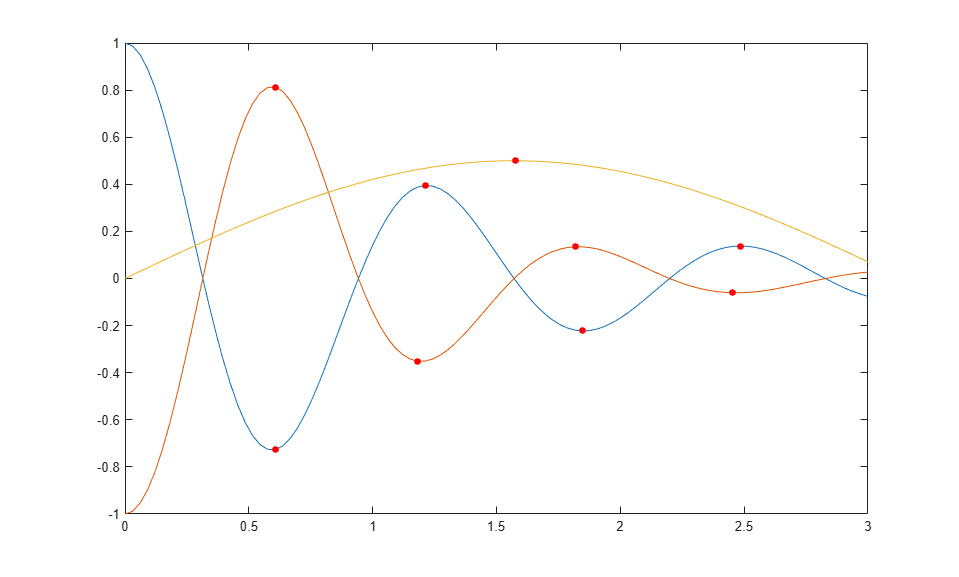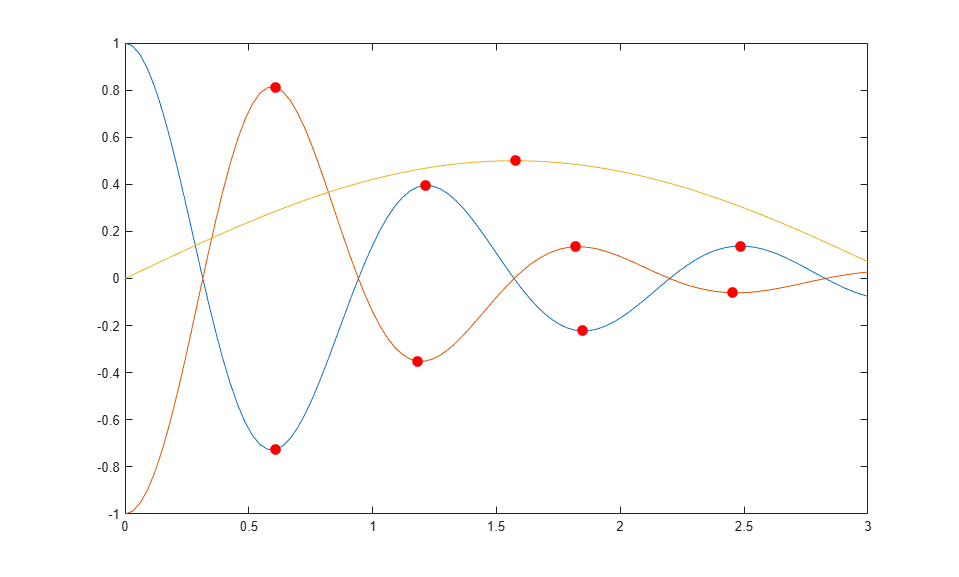# Chart Class with Variable Number of Lines

This example shows how to define a class of charts that can display any number of lines based on the size of the user's data. The chart displays as many lines as there are columns in the `YData` matrix. For each line, the chart calculates the local extrema and indicates their locations with circular markers. The following code demonstrates how to:

• Define two properties called `PlotLineArray` and `ExtremaArray` that store the objects for the lines and the markers, respectively.

• Implement an `update` method that replaces the contents of the `PlotLineArray` and `ExtremaArray` properties with the new objects. Because this method executes all the plotting and configuration commands, the `setup` method is empty.

You can use this example to become familiar with the coding techniques of chart development, or as the basis for a class you plan to develop.

To define the class, copy this code into the editor and save it with the name `LocalExtremaChart.m` in a writable folder.

```classdef LocalExtremaChart < matlab.graphics.chartcontainer.ChartContainer properties XData (1,:) double = NaN YData (:,:) double = NaN MarkerColor (1,3) double {mustBeGreaterThanOrEqual(MarkerColor,0),... mustBeLessThanOrEqual(MarkerColor,1)} = [1 0 0] MarkerSize (1,1) double = 5 end properties(Access = private,Transient,NonCopyable) PlotLineArray (:,1) matlab.graphics.chart.primitive.Line ExtremaArray (:,1) matlab.graphics.chart.primitive.Line end methods(Access = protected) function setup(~) end function update(obj) % get the axes ax = getAxes(obj); % Plot Lines and the local extrema obj.PlotLineArray = plot(ax,obj.XData,obj.YData); hold(ax,'on') % Replicate x-coordinate vectors to match size of YData newx = repmat(obj.XData(:),1,size(obj.YData,2)); % Find local minima and maxima and plot markers tfmin = islocalmin(obj.YData,1); tfmax = islocalmax(obj.YData,1); obj.ExtremaArray = plot(ax,newx(tfmin),obj.YData(tfmin),'o',... newx(tfmax),obj.YData(tfmax),'o',... 'MarkerEdgeColor',obj.MarkerColor,... 'MarkerFaceColor',obj.MarkerColor,... 'MarkerSize',obj.MarkerSize); hold(ax,'off') end end end ```

After saving the class file, you can create an instance of the chart. For example:

```x = linspace(0,3); y1 = cos(5*x)./(1+x.^2); y2 = -cos(5*x)./(1+x.^3); y3 = sin(x)./2; y = [y1' y2' y3']; c = LocalExtremaChart('XData',x,'YData',y); ```Change the marker size to `8`.

```c.MarkerSize = 8; ```